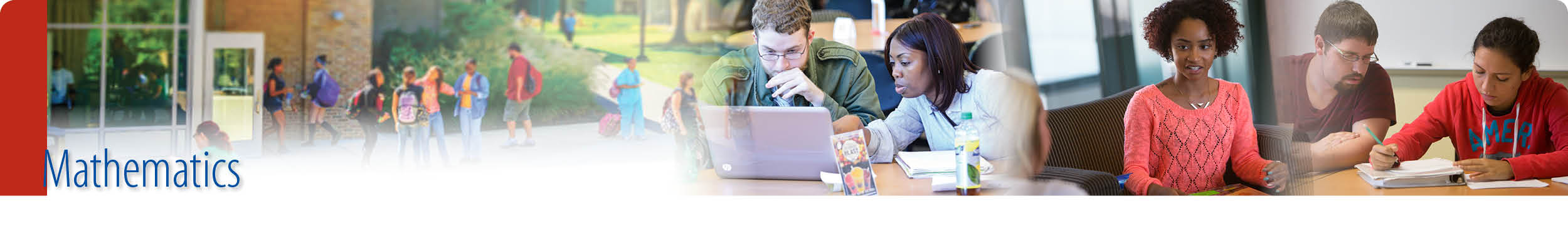## Math Department

.

Math Course Listing
• MATH 50 Preparation for College Mathematics
• MATH 67 Educator Preparation in Mathematics
• MATH 101 Foundations of Mathematics
• MATH 101A Foundations of Mathematics with Algebra
• MATH 110 Fundamental Concepts of Mathematics
• MATH 113 Fundamental Concepts of Mathematics II
• MATH 120 Elementary Statistics
• MATH 120A Elementary Statistics with Algebra
• MATH 127 Statistics With Probability
• MATH 145 College Algebra
• MATH 145S College Algebra with Support
• MATH 165 Precalculus
• MATH 170 Introduction to Discrete Mathematics
• MATH 175 Applied Calculus
• MATH 185 Calculus I
• MATH 195 Calculus II
• MATH 220 Intro to MATLAB©
• MATH 265 Linear Algebra
• MATH 275 Differential Equations
•  MATH285 Calculus III

## Contact Information

Jeanne Nesbitt
301.846.2530
​Office: B228

Kylena Cross
Department Chair
240.629.7835
Office: B230

### Related Information

Two Year Course Rotation

STEM Learning Center